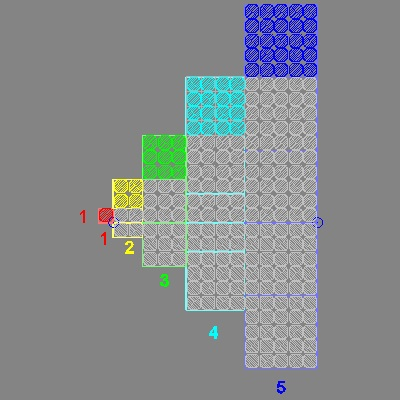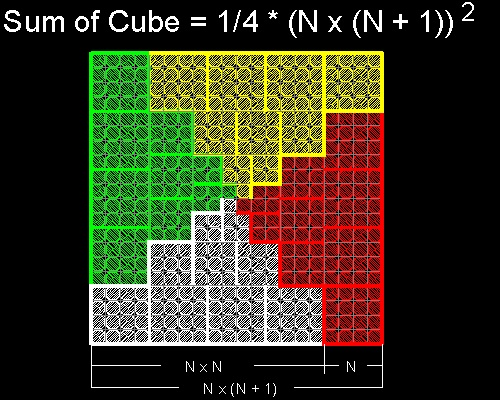Sum of integers cubed

### Sum of integers cubed

List of animations posted on this page.(Click the text to watch animation.)
Sum of cube - 1
Sum of cube - 2

### Fibonacci's elegant proof

(Ref. 2, 4)
Sum of odd integers,starting with 1, gives the square as shown in the diagram below.
```               1                              =  12
1 + 3                            =  22
1 + 3 + 5                          =  32
1 + 3 + 5 + 7                        =  42
1 + 3 + 5 + 7 + 9                      =  52
1 + 3 + 5 + 7 + 9 + 11                   =  62
```
One way to interpret this pattern is "the average number of the N-th row is N !!"
Therefore the sum of the row,which is the sum of odd integers, is NxN=N2.
This is what we has been discussed in the previous section on integer.

Fibonacci(1170-1250) arranged the same odd numbers in a different pattern as shown below
and came up with a very elegant proof regarding the sum of integers cubed.

```                1                             =  13
3 + 5                           =  23
7 + 9 + 11                        =  33
13 + 15 + 17 + 19                     =  43
21 + 23 + 25 + 27 + 29                  =  53
31 + 33 + 35 + 37 + 39 + 41                =  63
```
Fibonacci's interpretation of this table is the same as above.
"the average number of the N-th row is N2 !!"
So the N-th row has N terms and their average is N2.
Therefore the sum of the row is N2 x N = N3.

Now let us take a look at the left hand side.
The last term of the odd integer on the N-th row is a triangular number TN,
because TN = 1 + 2 + .. + N
And we know that sum of the first p odd integers is p2
Therefore Fibonacci concluded
13+ 23+ 33+ ... + N3 = (1 + 2 + 3 + ... + N)2 = (TN)2 = {(1/2)N(N+1)}2

### Method 2

(Ref. 1 ,3)
Compare the figure below with Fibonacci's diagram. The average numbers in Fibonacci's diagram
are now represented by squares in the diagonal position.You can see the process in animation for N=6 case.
It is easy to figure out the following relation.
13 + 23 + 33 + 43 + .. + N3 = (1 + 2 + 3 + 4 + .. + N)2

Tn = 1 + 2 + 3 + ... + n = (1/2)N(N+1)
So Sum of cube from 1 to N = (1/4){N(N+1)}2

To create this drawing and animation:
Then from command line, type sumcube_2

**************sumcube_2.dwg **************

Now let us separate upper left portion of this tile pattern as shown in the left below.
If this is rotated 90 degrees clock-wise, and aligned with the horizontal line,
where numbers 1,2,3,4 & 5 are written ,then we have a staircase like pattern
as shown in the right.
Just as the original square rotated 90 degrees around the red tile 4 times forms a square.
so does the staircase . This will lead to the next visualization scheme.*************sumcube_2_1.dwg ************ ************sumcube_2_2.dwg **************

### Method 3

Sum of integers cubed from 1 to N arranged in the staircase like pattern(Ref. 3),
which is then copied around 4 times, will form a square.
From the figure it is easy to see
4 x Sum = {N (N + 1)}2.
Therefore Sum = (1/4){N (N + 1)}2.You can see the process in animation
for N=4 case.

To create this drawing and animation:
Then from command line, type sumcube_1

*****************sumcube_1_final.dwg *****************

#### References

Go to   Fun_Math Content Table   Sums of Integers and Series

All comments/suggestions should be sent to Takaya Iwamoto

Last Updated July 9-th, 2006